Register for Maths, Science, English, Reasoning Olympiad Exams Register here | Book Free Demo for Live Olympiad Classes here | Check Olympiad Exam Dates here | Buy Practice Papers for IMO, IOM, HEO, IOEL etc here | Login here to participate in all India free mock test on every Saturday

# MensurationWhat is Mensuration? We all come across various shapes in our life which includes rectangles, squares, triangles and a lot more. But a very important question arises that how do we measure these shapes? What are their length, width and height and what area do they occupy? Here we are going to learn about the measurements of these various shapes.

Mensuration is basically nothing but the measurements of the dimensions, areas and various other parameters of any closed or open figure.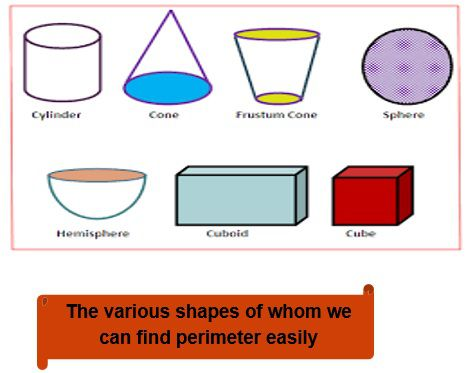Perimeter

Perimeter is the distance covered along the boundary forming a closed figure when you go round the figure once.

Perimeter has a great physical application which includes like

• Calculated perimeter is the length of fence required to surround garden or park.
• The perimeter of a wheel called as circumference describes how far it will roll in one revolution.
• The amount of string wound around a spool is related to the spool's perimeter.

Perimeter of a Rectangle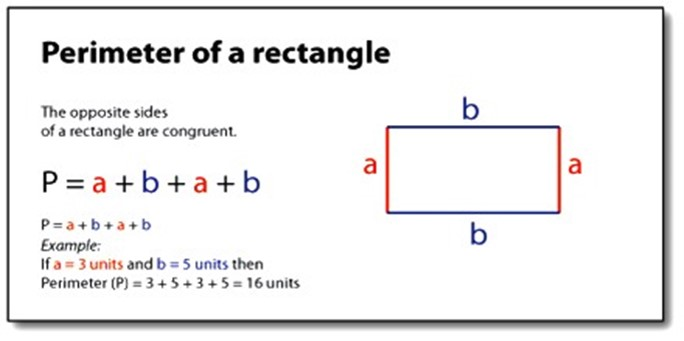Example 1: Find the perimeter of a regular pentagon whose side length is 5 cm.

Solution: No. of sides = 5

Length of each side = 5 cm.

Perimeter = 5+5+5+5+5 or 5 x 5 = 25 cm.

Hence the perimeter comes out to be 25 cm.

Perimeter of Square

= 4 x (Side of Square)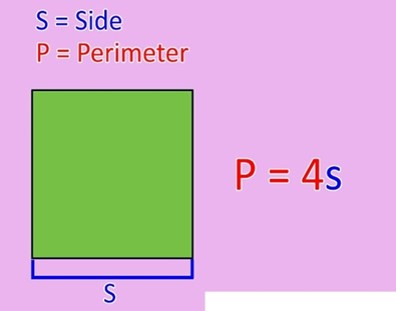Example 2: The perimeter of a square park is 20 cm. If it’s one side has to be fenced by a wire again, then find out the cost needed at a rate of Rs 4/cm.

Solution: Perimeter of Square = 20 cm = 4 x (Side of Square)

And from here we get side of square = 20cm / 4 = 5cm.

Hence the length of side required to be fenced = 5cm

Rate = Rs 4/cm

Therefore total cost = 5 x Rs 4 = Rs 20.

Perimeter of Triangle

3 x length of a side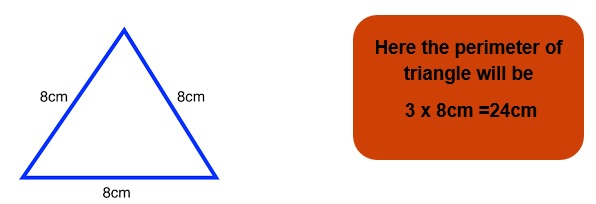Area

The amount of surface enclosed by a closed figure is called it’s area.

Area of Square = (Length of side)2

Area of Rectangle = Length x Breadth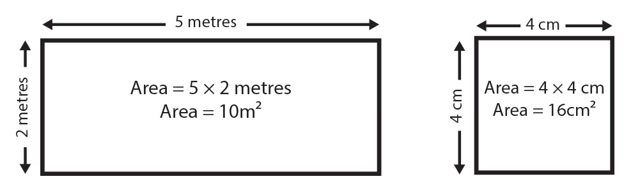Practice Questions

Q1) Find the perimeter of a rectangle of length 12 cm and breadth 10 cm.

Q2) If the perimeter of a triangle is 72 cm. Find the length of the side of the triangle.

Q3) Find the area and perimeter of a square of side 8 cm.

Q4) If the area of a rectangle is 20 cm2 and the perimeter is 18 cm. Then find the length and breadth of the rectangle.

Q5) A room has a dimension of 40cm x 20cm. If the rate of tiling the floor is Rs 100/cm2, then find the cost of tiling the floor.

Recap

• Perimeter is the distance covered along the boundary when you go round the boundary once.

• Perimeter of a rectangle = 2 × (length + breadth)
• Perimeter of a square = 4 × length of its side
• Perimeter of an equilateral triangle = 3 × length of a side

• The amount of surface enclosed by a closed figure is called its area.

• Area of a rectangle = length × breadth
• Area of a square = side × side

The figures in which all sides and angles are equal are called regular closed figures.

## Quiz for Mensuration

 Q.1 When a 576 cm straight wire used to make an octagon. What would be the length of its each side? a) 65cm b) 70cm c) 72cm d) 80cm
 Q.2 Ratio of length to breadth of a rectangle is 7:5. If the area of the rectangle is 875 sq cm, find the difference between length and breadth. a) 10cm b) 20cm c) 5cm d) 2cm
 Q.3 If 32 square marble tiles of side 20cm are used to cover a square floor and 30 rectangular tiles of sides 22 cm by 18cm are used to cover a rectangular floor, find the difference between the area of square and the rectangular floor. a) 500 sq cm b) 650 sq cm c) 780 sq cm d) 920 sq cm
 Q.4 If length of each side of a square doubles, its area will increase by how many times? a) 2 b) 4 c) 6 d) 8
 Q.5 If length of a rectangle becomes double and breadth of that rectangle reduces to its half at the same time, its area will increase by how many times? a) 2 b) 4 c) Remains same d) 6
 Q.6 A student is making 2 paper rectangles. One has its sides 15cm by 13cm and other has 18cm by 12cm. He will keep smaller rectangle on the surface of bigger rectangle. Then he starts to color them but he left the common portion of both rectangles and colors rest part. How much area will be colored? a) 20 sq cm b) 21 sq cm c) 30 sq cm d) 29 sq cm
 Q.7 Find perimeter of the given shape.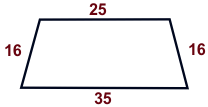a) 92 unit b) 102 unit c) 82unit d) 62 unit
 Q.8 Find perimeter of the given shape.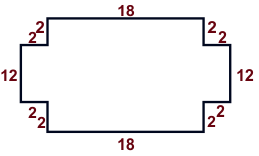a) 86 unit b) 56 unit c) 52 unit d) 76 unit
 Q.9 Find perimeter of the given shape.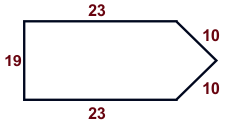a) 78 unit b) 85 unit c) 98 unit d) 67 unit
 Q.10 Find perimeter of the shape.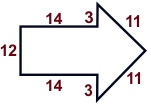a) 68 unit b) 89 unit c) 47 unit d) 65 unit